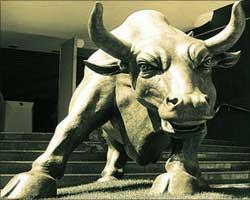Rediff.com  » Business » Investing in stocks: How 'moving averages' help

Investing in stocks: How 'moving averages' help

July 27, 2009 16:10 ISTMoving averages are a simple and yet very effective tool. These smoothen the data in volatile times and makes trend spotting easier. A simple moving average is formed by computing the average price of a security over a specified number of periods.

To make matters simple, let us calculate a five-day moving average for a stock. If a stock has closed at Rs 10, 30, 50, 40 and 20, respectively, for the past five sessions, its five-day average would be 30.

What happens on the sixth day, when it closes at 30? The five-day closing prices are now 30, 50, 40, 20 and 30. The new average is 35.

How many days?

The number of periods used in a moving average will vary according to the security's volatility and personal preferences. The more volatility there is, the more smoothing that will be required and, hence, the longer the moving average. Some of the popular parameters for moving averages include 8-day and 21-day, while longer-term investors may prefer 50, and 200 DMA. Examine how the moving average fits with the price data of the security you follow. If there are too many breaks, lengthen the moving average to decrease its sensitivity. If the moving average is slow to react, shorten it to increase its sensitivity.

Functions

There are three basic uses:

• Trend identification and confirmation
• Support and Resistance level identification/confirmation
• Identify Entry and Exit points

Trends

There are three ways to identify the trend with moving averages. The first uses the direction of the moving average. If the moving average is rising, the trend is  up and if the moving average is declining, the trend is considered down. The direction of a moving average can be observed by looking at its plot.

The second technique for trend identification is price location. The location of the price relative to the moving average can be used to determine the basic trend. If the price is above the moving average, the trend is considered up. If the price is below the moving average, the trend is considered down.

The third technique for trend identification is based on the crossover of the shorter moving average (50-day) relative to the longer (200-day) moving average. If the shorter moving average is above the longer moving average, the trend is considered up. If the shorter moving average is below the longer moving average, the trend is considered down.

For example, if 20 DMA crosses 50 DMA upside, then a new uptrend may emerge. The opposite also holds true, as the short moving average crosses down and below the long moving average, a new downtrend may emerge.

Support/resistance

Another use of moving averages is to identify support and resistance levels. This is usually accomplished with one moving average and is based on historical precedent. Support and resistance level identification through moving averages works best in trending markets. 20, 50, and 200 days' moving average are the most used parameters.

Exponential (EMA)

To reduce the lag in simple moving averages, technicians often use exponential moving averages. Exponential Moving Averages reduce the lag by applying more weight to recent prices, relative to older prices. The weighting applied to the most recent price depends on the specified period of the moving average. The shorter the EMA's period, the more weight will be applied to the most recent price. As such, EMAs will react quicker to recent price changes.

Moving averages follow stock prices like a shadow. They help in determining the underlying trend in prices. We all know stock prices are volatile and it sometimes become difficult to judge the actual direction. The moving average removes clutter and gives an unbiased opinion.

The writer is director and head of research, Anagram Capital

Vinod Sharma